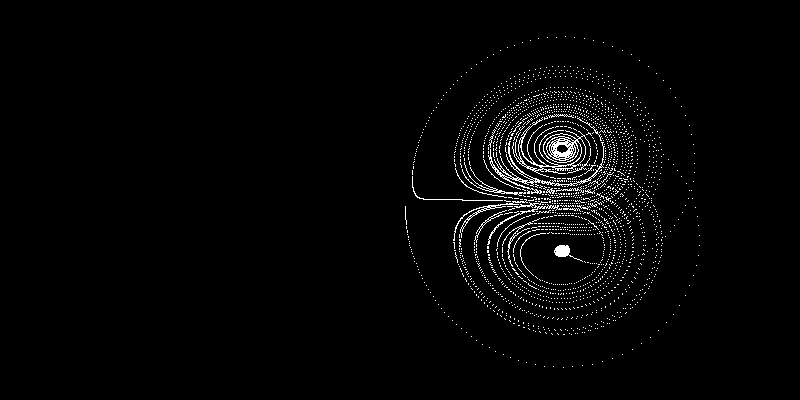# Lorenz Attractor

### Rendered in Python, explained in English

##### By Dany Shaanan
A Lorenz Attractor is a solution of the Lorenz equations, which are ordinary differential equations in 3D. Some exhibit chaotic behavior and create nice looking patterns. The Lorenz differential equations are:
```dx/dt = o * (y - x)
dy/dt = x * (p - z) - y
dz/dt = x * y - b * z
```
These equations describe how, at each point in 'time', x, y and z affect the direction of the attractor. Once we move a bit in that direction, x, y and z change, and so does the direction. In order to simulate this without solving the differential equation, we iterate over small enough timesteps.

To pick a specific set of Lorenz equations, we'll set the euqations constants to:
```o = 10
p = 28
b = 8/3
```
Showing the 3D attractor on a 2D image will not let us see its 3D complexity, but an animation of it turning around the y axis can let us see just that:

See the code here